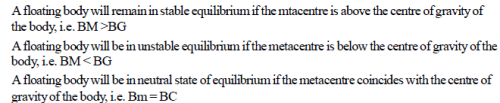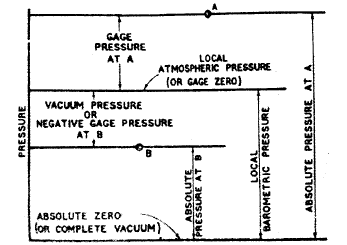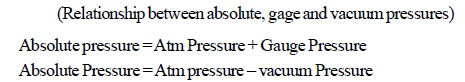Courses

# Test: Fluid Mechanics Level - 2

## 25 Questions MCQ Test Civil Engineering SSC JE (Technical) | Test: Fluid Mechanics Level - 2

Description
This mock test of Test: Fluid Mechanics Level - 2 for Civil Engineering (CE) helps you for every Civil Engineering (CE) entrance exam. This contains 25 Multiple Choice Questions for Civil Engineering (CE) Test: Fluid Mechanics Level - 2 (mcq) to study with solutions a complete question bank. The solved questions answers in this Test: Fluid Mechanics Level - 2 quiz give you a good mix of easy questions and tough questions. Civil Engineering (CE) students definitely take this Test: Fluid Mechanics Level - 2 exercise for a better result in the exam. You can find other Test: Fluid Mechanics Level - 2 extra questions, long questions & short questions for Civil Engineering (CE) on EduRev as well by searching above.
QUESTION: 1

Solution:
QUESTION: 2

Solution:
QUESTION: 3

### Alcohol is used in manometers because

Solution:
QUESTION: 4

Free surface of a liquid tends to contract to the smallest possible area due to force of

Solution:
QUESTION: 5

Falling drops of water become spheres due to the property of

Solution:
QUESTION: 6

If cohesion between molecules of a fluid is greater than adhesion between fluid and glass, then the free level of fluid in a dipped glass tube will be

Solution:
QUESTION: 7

The point in the immersed body through which the resultant pressure of the liquid may be taken to act is known as

Solution:
QUESTION: 8

The resultant upward pressure of the fluid on an immersed body is called

Solution:
QUESTION: 9

The conditions for the stable equilibrium of a floating body are

Solution:QUESTION: 10

Poise is the unit of

Solution:
QUESTION: 11

The buoyancy depends on

Solution:
QUESTION: 12

The centre of gravity of the volume of the liquid displaced by an immersed body is called

Solution:
QUESTION: 13

A piece of metal of specific gravity 13.6 is placed in mercury of specific gravity 13.6, what fraction of it volume is under mercury

Solution:
QUESTION: 14

The capillary rise at 20ºC in a clean glass tube of 1 mm bore containing water is approximately

Solution:
QUESTION: 15

If the surface of liquid is convex, then

Solution:
QUESTION: 16

To avoid an interruption in the flow of a syphon, an air vessel is provided

Solution:
QUESTION: 17

The vapour pressure over the concave surface is

Solution:
QUESTION: 18

The process of diffusion of one liquid into the other through a semi-permeable membrane is called

Solution:
QUESTION: 19

The dimensions of coefficient of viscosity are

Solution:
QUESTION: 20

The continuity equation is connected with

Solution:
QUESTION: 21

Capillary action is due to the

Solution:
QUESTION: 22

Newton's law of viscosity is a relationship between

Solution:
QUESTION: 23

The atmospheric pressure with rise in altitude decreases

Solution:QUESTION: 24

Barometer is used to measure

Solution:
QUESTION: 25

Piezometer is used to measure

Solution: